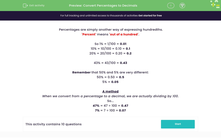# Convert Percentages to Decimals

In this worksheet, students must convert the given percentages into their decimal equivalents.Key stage:  KS 2

Curriculum topic:   Number: Fractions, Decimals and Percentages

Curriculum subtopic:   Know Fraction-Decimal-Percentage Equivalents

Popular topics:   Fractions worksheets, Percentages worksheets

Difficulty level:#### Worksheet Overview

Percentages are simply another way of expressing hundredths.

'Percent' means 'out of a hundred'.

So 1% = 1/100 = 0.01

10% = 10/100 = 0.10 = 0.1

20% = 20/100 = 0.20 = 0.2

43% = 43/100 = 0.43

Remember that 50% and 5% are very different:

50% = 0.50 = 0.5

5% = 0.05

A method

When we convert from a percentage to a decimal, we are actually dividing by 100.

So...

47% = 47 ÷ 100 = 0.47

7% = 7 ÷ 100 = 0.07

### What is EdPlace?

We're your National Curriculum aligned online education content provider helping each child succeed in English, maths and science from year 1 to GCSE. With an EdPlace account you’ll be able to track and measure progress, helping each child achieve their best. We build confidence and attainment by personalising each child’s learning at a level that suits them.

Get started